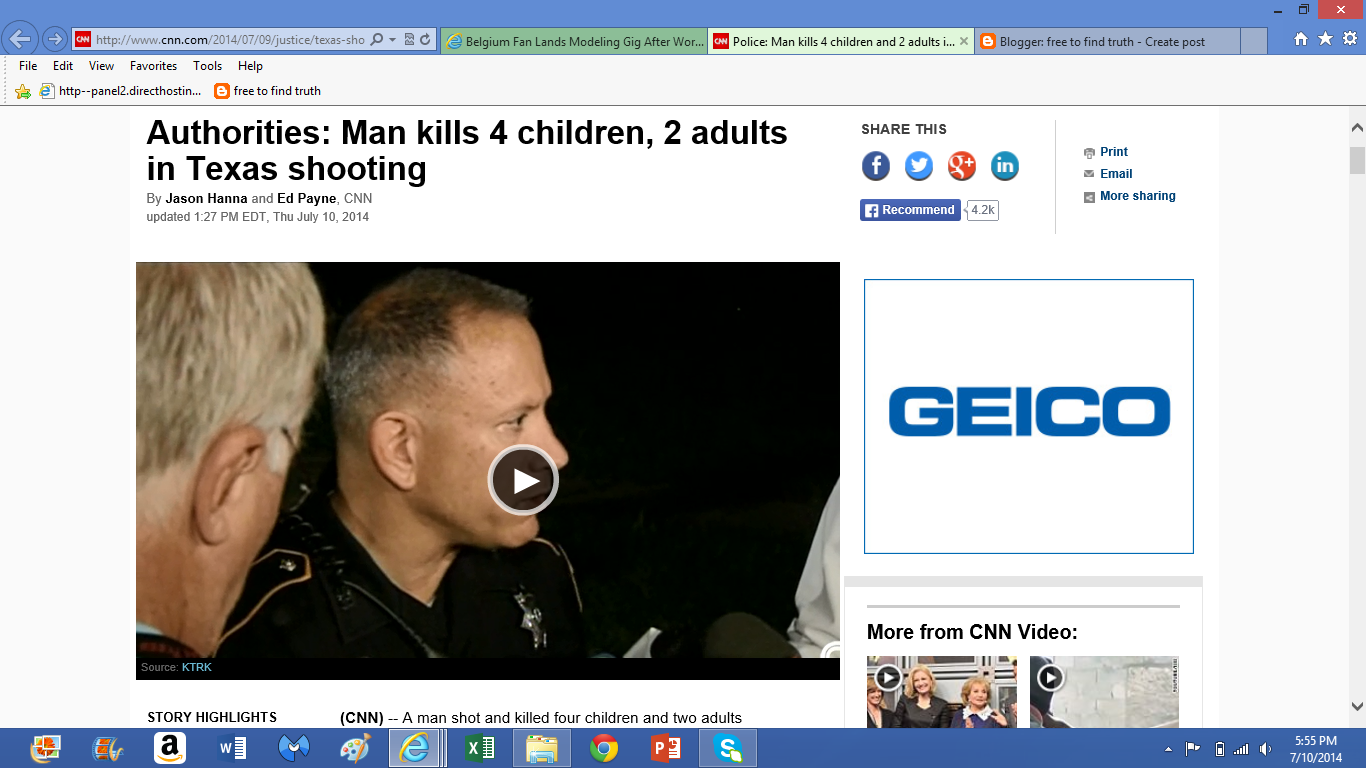## Thursday, July 10, 2014

### 33 | Ron Lee Haskell Murder Spree, July 9, 2014Jason Hanna = 1+1+1+6+5+8+1+5+5+1 = 34 | Ed Payne = 5+4+7+1+7+5+5 = 34
Today it is reported that 34-year old Ron Lee Haskell went into the home of family and killed the two parents and four children.  A reported seventh person was hurt and in the hospital.  Let us examine the Gematria of this story that is very deliberately coded with 33.
• Ron Lee = 9+6+5+3+5+5 = 33
• Haskell = 8+1+1+11+5+3+3 = 32
• Ron Lee Haskell = 33+32 = 65, reduces to 11
• The names Ron and Lee both have master numerology alone
• Ron = 18+15+14 = 47, reduces to 11
• Lee = 12+5+5 = 22
The victims of his "rampage" are as follows; Stephen Stay, 39; Katie Stay, 33; two boys, ages 13 and 4; and two girls, ages 9 and 7, the Harris County Sheriff's Office said
• Stephen 1+2+5+7+8+5+5 = 33
• Katie = 11+1+2+9+5 = 28
• Ages "13 and 4"; 13 = 1+3 + 4; thus 4 and 4 or 44
• 44 is associated with murder/assassination
• Kill = 11+9+12+12 = 44
• If you add up the ages 4, 7, 9 and 13, you get 33.
• 4+7+9+13 = 33
• Stay = 1+2+1+7 = 11
• Stephen Stay = 44
• Katie Stay = 39
• Children's names not released, just ages
• Stepehen Stay was 39
• Katie Stay was 33
In other words, this story is yet another hoax of numbers- all for the purpose of the negative psychological tole it takes on the millions of Americans who catch wind of it.  What a sham!  What a shame!  Above I mentioned "44" being connected to assassination.  In this story, this sham, it is reported that the gunman dressed up as a "FedEx" employee.
• FedEx = 6+5+4+5+24 = 44
• Federal Express...
• Federal = 6+5+4+5+9+1+3 = 33
Police Officer Hickman said this was the worst tragedy he has seen in "over 40-years of service".
• Police = 7+6+3+9+3+5 = 33
• Hickman = 8+9+3+11+4+1+5 = 41